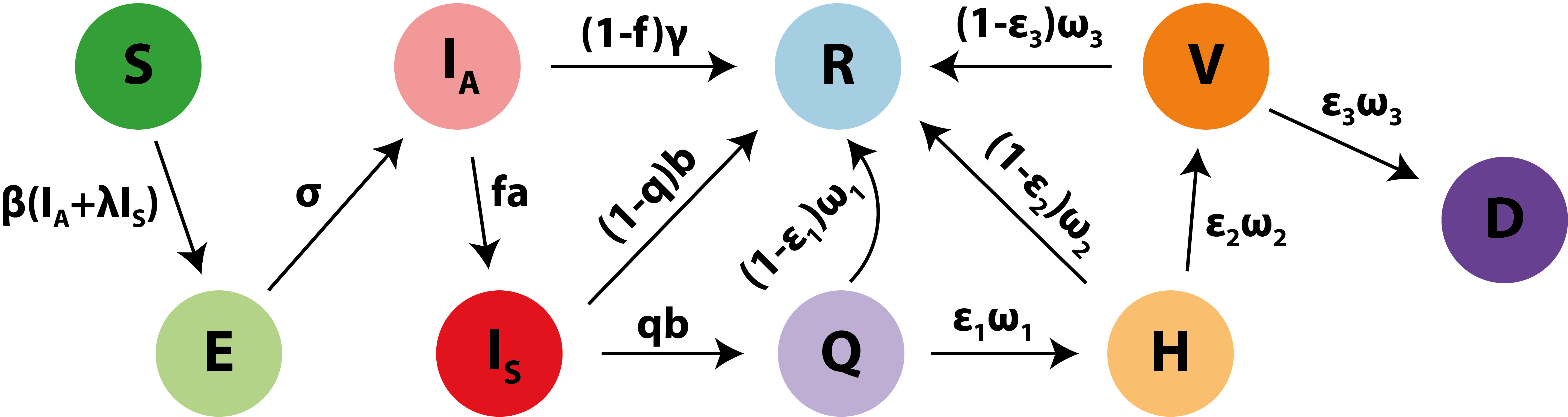# Real-time analysis of the COVID-19 pandemic in Poland

## Methodology

The underlying epidemiological model is based on the classical SEIR approach with the important difference that it explicitly considers people who are admitted to the hospital, the ICU and those who die from infection. The exact model is a deterministic version of the epidemiological model considered in the Report 9 of the MRC Centre for Global Infectious Disease Analysis at Imperial College London . The model, summarised in the Figure below, makes several important simplifications/assumptions. First, it does not consider the population demography and assumes homogeneous mixing. Second, tt assumes that all COVID-related deaths occur at ICU and are reported immediately. I also assume that quarantined, hospitalised and ICU patients are isolated well enough to not be infectious anymore.Figure. Summary of the epidemiological model used. Susceptibles (S) become exposed (E) when they get in touch with an infectious person, either asymptomatic ($$I_A$$) or symptomatic ($$I_S$$), at a rate $$\beta(I_A+\lambda I_S)$$, where $$\beta$$ is a transmission rate and $$\lambda$$ reflects relative contribution of a symptomatic person to transmission compared to an asymptomatic person. An asymptomatically infected person can then develop symptoms with a probability $$f$$ at a rate $$a$$ or otherwise recover (R) at a rate $$\gamma$$. A symptomatic person can become seriously enough ill to undergo self-isolation (Q) with a probability $$q$$ at a rate $$b$$, such that $$1/a+1/b=1/\gamma$$. A proportion $$\epsilon_1$$ of quarantined individuals become hospitalised (H) at a rate $$\omega_1$$, otherwise recover at a rate $$(1-\epsilon_1)\omega_1$$. A proportion $$\epsilon_2$$ of hospitalised individuals become admitted to the ICU (V) at a rate $$\omega_2$$, otherwise recover at a rate $$(1-\epsilon_2)\omega_2$$. A proportion $$\epsilon_3$$ of ICU patients die (D) at a rate $$\omega_3$$, otherwise recover at a rate $$(1-\epsilon_3)\omega_3$$. In this model, $$\beta=R_0\gamma/N\frac{1+fa/b}{1+\lambda fa/b}$$, where $$R_0$$ is the basic reproduction number and $$N$$ is the population size. The epidemic begins at time $$t_{case}-T_{seed}$$, where $$t_{case}$$ is the date of the first reported case (04/03) and $$T_\text{seed}$$ is the period of time between the first infection and the first actual case. Governmental lockdowns were introduced between March 12th and 16th, and I assume 16/03 to be the date of lockdown introductiona. After this the transmission of the virus is assumed to be $$R=\kappa R_0$$, where $$\kappa\in[0,1]$$ reflects the relative reduction in $$R_0$$ due to the lockdowns and $$R$$ is the effective transmission coefficient.

I used the existing literature to make assumptions about the parameters used in the model above.

Parameter Explanation Value Comment Source
$$N$$ Population size of Poland 38 386 000 
$$1/\sigma$$ latent period (average time from exposure to infectiousness) 4.6 days 
$$1/a$$ average time from infectiousness to onset of symptoms 0.5 day $$1/\sigma+1/a$$ is incubation period of 5.1 days [1,3,4]
$$1/b$$ average time from onset to recovery/quarantine 1.4 days based on assumed generation time of 6.5 days 
$$1/\gamma$$ mean infectious period 1.9 days equals to $$1/a+1/b$$ 
$$f$$ Proportion of infected patients who become symptomatic 80% 
$$q$$ Proportion of cases which are sufficiently symptomatic to self-isolate 2/3 
$$1/\omega_1$$ Average time spent in quarantine 3.6 days Assumes mean time of 5 days from onset to hospital admission 
$$1/\omega_2$$ Average time spent in hospital 8 days 
$$1/\omega_3$$ Average time spent at ICU 8 days Assumes mean time of 21 days from onset to death/discharge [1,6]
$$\epsilon_1$$ Proportion of quarantined cases requiring hospitalisation 6.6% Assumes that 4.4% of symptomatic infections are hospitalised 
$$\epsilon_2$$ Proportion of hospitalised cases that end up at ICU 30% 
$$\epsilon_3$$ Proportion ICU cases that result in death 57% Tailored to reflect the infection fatality-rate of about 0.9% [1,5]
$$\lambda$$ Relative transmission of a symptomatic person compared to asymptomatic person 2 Assumes that symptomatic people are twice as infectious as asymptomatic ones 
$$R_0$$ Basic reproduction number (see here for explanation) Estimated
$$\kappa$$ Efficacy of governmental lockdowns Estimated
$$T_\text{seed}$$ Time from seeding the epidemic to the first reported case Estimated

Table 1. Parameters of the COVID-19 transmission model. The model is fit to the data using a maximum-likelihood approach by comparing the predicted to the reported number of deaths. The daily number of deaths is assumed to be Poisson distributed. Using the parameter values assumed above, we estimate the values of $$R_0$$, $$T_\text{seed}$$ and $$\kappa$$ that best explain the observed data given the assumed model.